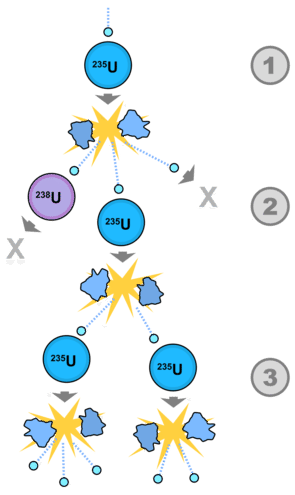# 24.6: Nuclear Fission Processes

Nuclear fission was first discovered by two German scientists, Fritz Strassman and Otto Hahn, in the 1930's. They began their work by bombarding uranium with neutrons, hoping to create larger elements. Instead, they were very surprised to find $$\ce{Ba}$$-141, a much smaller element. They immediately contacted a fellow scientist in the field, Lise Meitner, who carried out calculations to demonstrate that fission had taken place.

## Nuclear Fission

Radioactive decay by the release of alpha or beta particles is not the only way new isotopes are formed. When a neutron collides with a nucleus the nucleus splits into two isotopes, each of which is roughly half the mass of the original atom. A small amount of mass is "left over" and released as energy, as predicted by Einstein's famous equation $$E = mc^2$$, that relates mass and energy. This process is known as nuclear fission. The neutron must be a "slow" neutron, traveling at a speed that is approximately that of the molecules of a gas at the same temperature in the system producing the neutrons. High-speed ("fast") neutrons will not result in nuclear fission.Figure 24.6.1: Fission of a uranium nucleus produced by collision with a neutron.

The example above illustrates the basic nuclear fission process. A neutron (generally produced by some controlled process, not usually a natural event) collides with an atom of $$\ce{U}$$-235. Momentarily, a $$\ce{U}$$-236 atom forms which then splits into two smaller atoms ($$\ce{Kr}$$-93 and $$\ce{Ba}$$-141) in the diagram. This process results in the release of three new neutrons, which can then initiate fission reactions with more atoms. We will see later how this propagation of neutrons can be employed in a reactor for the generation of electricity.

An extended version of this process can be seen in the figure below. Not every collision of a neutron with $$\ce{U}$$-235 results in a fission reaction. A neutron from the initial fission process may strike an atom of $$\ce{U}$$-238, which does not continue the process. Another neutron may not collide with a nucleus and is lost in the environment. However, a third neutron produced from the initial collision can collide with more $$\ce{U}$$-235 and continue the chain reaction to produce more neutrons.Figure 24.6.2: Fission reaction with $$\ce{U}$$-235.

Typical nuclear fission reaction balance in terms of mass. The total mass of the reactants is equal to the total mass of the products:

$\ce{^{235}_{92}U} + \ce{^1_0n} \rightarrow \ce{^{92}_{36}Kr} + \ce{^{142}_{56}Ba} + 2 \ce{^1_0n} + \text{energy}$

There are a total of 236 mass units on the left of the equation and 236 mass units on the right. In the same manner, we see 92 protons on the left and 92 on the right. The energy that is released is the binding energy that holds the nucleus together.

Another set of fission products from $$\ce{U}$$-235 can be seen in the following reaction:

$\ce{^{235}_{92}U} + \ce{^1_0n} \rightarrow \ce{^{95}_{42}Mo} + \ce{^{139}_{57}La} + 2 \ce{^1_0n} + \text{energy}$

Again we see that the total number of mass units and of protons is equal on both sides of the equation.

## Summary

• The process of nuclear fission is described.
• Examples of nuclear fission processes are illustrated.

## Contributors

• CK-12 Foundation by Sharon Bewick, Richard Parsons, Therese Forsythe, Shonna Robinson, and Jean Dupon.